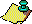Contents Next: Do divergent trajectories Up: The 3x+1 problem. Previous: Behavior of the

A first observation is that there are other cycles if negative integers are allowed in the domain of the function. There is a cycle of period 1 starting from n = -1, and there are cycles of length 3 and 11 starting from n = -5 and n = -17, respectively. Böhm and Sontacchi  conjecture that these cycles together with the cycles starting with n = 0 and n = 1 make up the entire set of cycles occurring under iteration of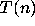applied to the integers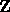. Several authors have proposed the following conjecture (, , , ).

### FINITE CYCLES CONJECTURE.

There are only a finite number of distinct cycles for the functioniterated on the domain.

One can easily show that for any given length k there are only a finite number of integers n that are periodic under iteration by T with period k, in fact at most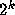such integers, as observed by Böhm and Sontacchi . To see this, substitute the equation (2.4) into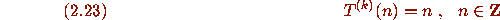to obtain the equation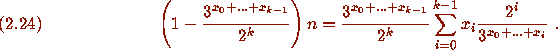There are onlychoices for the 0-1 vector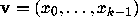, and for each choice ofthe equation (2.24) determines a unique rational solution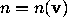. Consequently there are at mostsolutions to (2.23). Böhm and Sontacchi also noted that this gives an (inefficient) finite procedure for deciding if there are any cycles of a given length k, as follows: Determine the rational number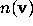for each of thevectors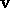, and for eachwhich is an integer test if (2.23) holds.

The argument of Böhm and Sontacchi is a very general one that makes use only of the fact that the necessary condition (2.24) for a cycle has a unique solution when the values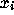are fixed. In fact, considerably more can be proved about the nonexistence of nontrivial cyclic trajectories using special features of the necessary condition (2.24). For example, several authors have independently found a much more efficient computational procedure for proving the nonexistence of nontrivial cyclic trajectories of period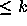; it essentially makes use of the inequality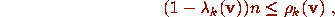which must hold for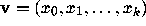satisfying (2.24). This approach also allows one to check the truth of the Coefficient Stopping Time Conjecture for all n with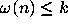. The basic result is as follows.

### Theorem H

(Terras). For each k there is a finite bound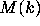given by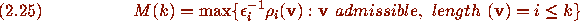such thatimplies that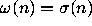whenever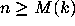. Consequently:
(i) If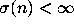for all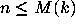, then there are no non-trivial cycles of length.
(ii) Iffor all, thenimplies.

### [Proof]

Theorem H can be used to show the nonexistence of nontrivial cycles of small period by obtaining upper bounds for theand checking that condition (i) holds. This approach has been taken by Crandall , Garner , Schuppar  and Terras . In estimating, one can show that the quantities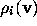are never very large, so that the size ofis essentially determined by how large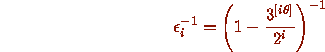can get. The worst cases occur when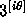is a very close approximation to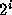, i.e., when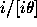is a very good rational approximation to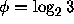. The best rational approximations to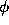are given by the convergents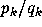of the continued fraction expansion of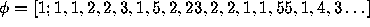. Crandall  uses general properties of continued fraction convergents to obtain the following quantitative result.

### Theorem I

(Crandall). Let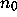be the minimal element of a purely periodic trajectory of period k. Then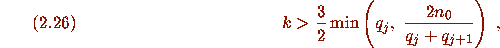where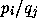is any convergent of the continued fraction expansion of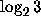with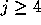.

As an application, use Yoneda's bound  that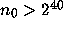and choose j = 13 in (2.26), noting that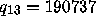and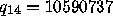, to conclude that there are no nontrivial cycles with period length less than 275,000.

Further information about the nonexistence of nontrivial cyclic trajectories can be obtained by treating the necessary condition (2.24) as an nonexponential Diophantine equation. Davidson  calls a purely periodic trajectory of period k a circuit if there is a value i for which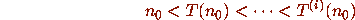and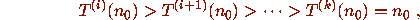i.e., the parity vectorhas the special form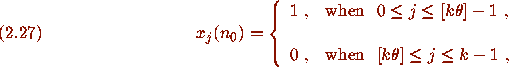where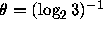. The cycle starting with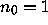is a circuit. Davidson observed that each solution to the exponential Diophantine equation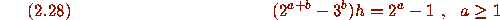gives rise to a circuit of length k = a + b with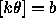and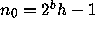, and conversely. (The equation (2.28) is the necessary condition (2.24) specialized to the vector (2.27). R. Steiner  showed that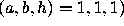is the only solution of (2.28), thus proving the following result.

### Theorem J

(Steiner). The only cycle that is a circuit is the trivial cycle.

### [Proof]

The most remarkable thing about Theorem J is the weakness of its conclusion compared to the strength of the methods used in its proof. The proof of Theorem J does have the merit that it shows that the coefficient Stopping Time Conjecture holds for the infinite set of admissible vectorsof the form (2.27).Contents Next: Do divergent trajectories Up: The 3x+1 problem. Previous: Behavior of the